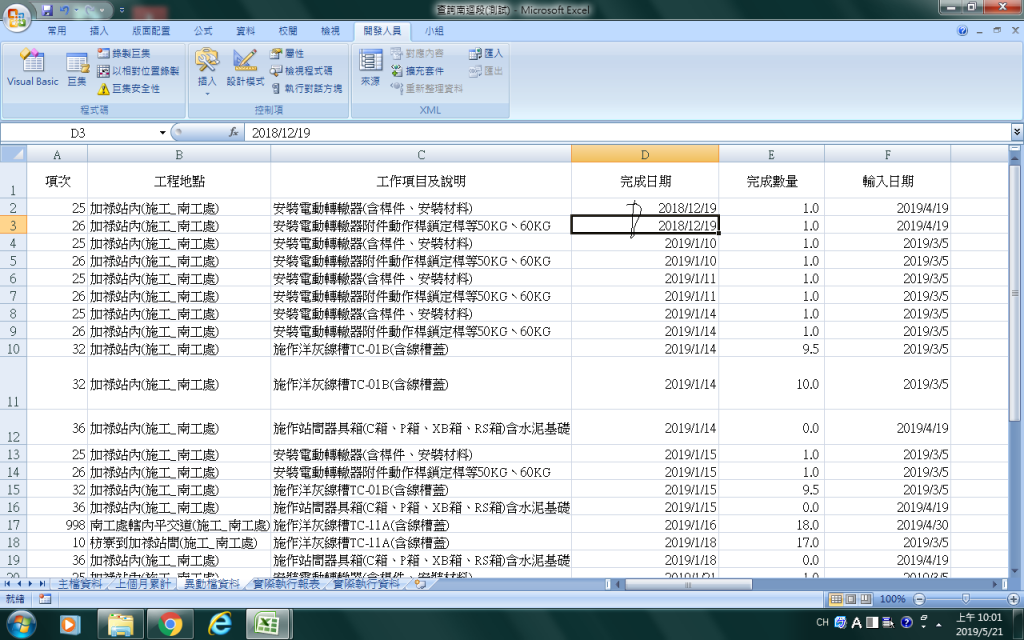#0

## VBA 2個日期範圍查詢``````'請問我程式這樣寫卻沒反應
Dim datestar, dateend As String  '定義2個字串變數
lastrow = ActiveSheet.UsedRange.Rows.Count + ActiveSheet.UsedRange.Rows(1).Row - 1  '最後一行
Worksheets("異動檔資料").Activate   '指定工作表
datestar = InputBox("要查詢開始日期的資料")  '輸入值給變數1
For j = lastrow To 2 Step -1    '迴圈
Val (datestar)  '把輸入的字串轉成數字
If datestar < Cells(j, "D") Then  '判斷小於開始日期
Rows(j).Delete    '若判斷成立就刪除
End If
Next      '下一個
dateend = InputBox("要查詢結束日期的資料")   '輸入值給變數2
For j = lastrow To 2 Step -1   '迴圈開始
val (dateend)    '把輸入的字串轉成數字
If Cells(j, "D") > dateend Then    '判斷大於結束日期
Rows(j).Delete            '若判斷成立就刪除ˊ
End If
Next   '下一個!

``````
goodnight iT邦研究生 3 級 ‧ 2019-05-21 13:43:41 檢舉

Dim datestart as variant, dateend as variant
lastrow = ActiveSheet.UsedRange.Rows.Count + ActiveSheet.UsedRange.Rows(1).Row - 1
Worksheets("異動檔資料").Activate '指定工作表
datestar = InputBox("要查詢開始日期的資料") '輸入值給變數1
dateend = InputBox("要查詢結束日期的資料") '輸入值給變數2

datestar = datevalue(datestar) '把輸入的字串轉成數字
dateend = datevalue(dateend) '把輸入的字串轉成數字

For j = lastrow To 2 Step -1 '迴圈
If datestar < Cells(j, "D") OR Cells(j, "D") > dateend Then '判斷小於開始日期
Rows(j).Delete '若判斷成立就刪除
End If
Next '下一個!

VBA告訴我型態錯誤

### 1 個回答

1
``````Dim datestart as variant, dateend as variant
lastrow = ActiveSheet.UsedRange.Rows.Count + ActiveSheet.UsedRange.Rows(1).Row - 1
Worksheets("異動檔資料").Activate   '指定工作表
datestar = InputBox("要查詢開始日期的資料")  '輸入值給變數1
For j = lastrow To 2 Step -1    '迴圈
datestar = datevalue(datestar)  '把輸入的字串轉成數字
If datestar < Cells(j, "D") Then  '判斷小於開始日期
Rows(j).Delete    '若判斷成立就刪除
End If
Next      '下一個
dateend = InputBox("要查詢結束日期的資料")   '輸入值給變數2
For j = lastrow To 2 Step -1   '迴圈開始
dateend = datevalue(dateend)    '把輸入的字串轉成數字
If Cells(j, "D") > dateend Then    '判斷大於結束日期
Rows(j).Delete            '若判斷成立就刪除ˊ
End If
Next   '下一個!
``````

VBA告訴我型態錯誤 我才把他改成字串再轉數字